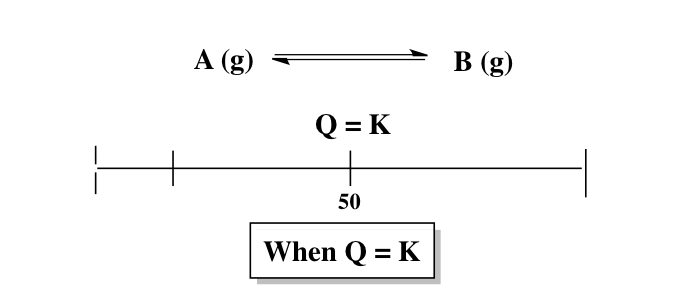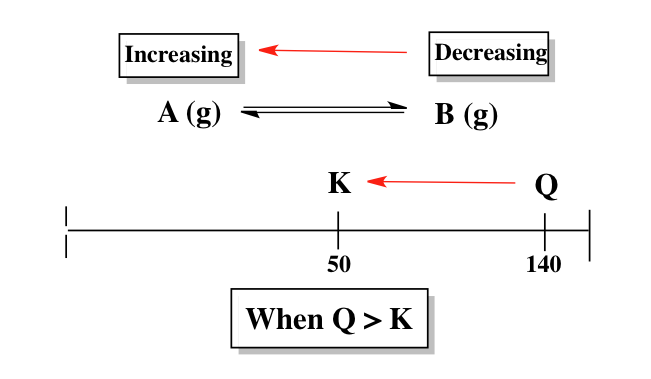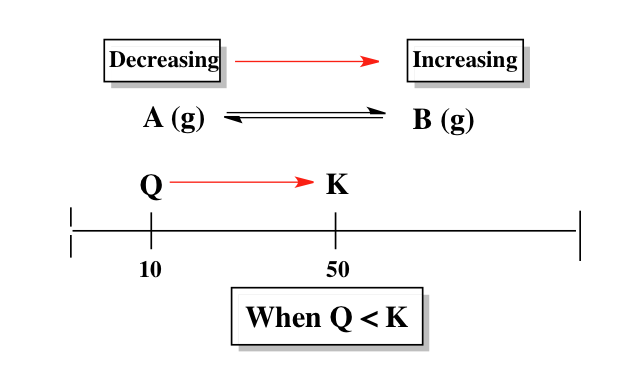Ch.6 - Chemical EquilibriumWorksheetSee all chapters
 Ch.1 - Chemical Measurements 2hrs & 10mins 0% complete Worksheet Ch.2 - Tools of the Trade 1hr & 26mins 0% complete Worksheet Ch.3 - Experimental Error 1hr & 51mins 0% complete Worksheet Ch.4 + 5 - Statistics, Quality Assurance and Calibration Methods 2hrs & 1min 0% complete Worksheet Ch.6 - Chemical Equilibrium 3hrs & 43mins 0% complete Worksheet Ch.7 - Activity and the Systematic Treatment of Equilibrium 1hr & 1min 0% complete Worksheet Ch.8 - Monoprotic Acid-Base Equilibria 1hr & 52mins 0% complete Worksheet Ch.9 - Polyprotic Acid-Base Equilibria 2hrs & 16mins 0% complete Worksheet Ch.10 - Acid-Base Titrations 2hrs & 22mins 0% complete Worksheet Ch.11 - EDTA Titrations 1hr & 37mins 0% complete Worksheet Ch.12 - Advanced Topics in Equilibrium 1hr & 18mins 0% complete Worksheet Ch.13 - Fundamentals of Electrochemistry 2hrs & 27mins 0% complete Worksheet Ch.14 - Electrodes and Potentiometry 55mins 0% complete Worksheet Ch.15 - Redox Titrations 1hr & 14mins 0% complete Worksheet Ch.16 - Electroanalytical Techniques 1hr & 23mins 0% complete Worksheet Ch.17 - Fundamentals of Spectrophotometry 55mins 0% complete Worksheet BONUS: Chemical Kinetics Not available yet

# The Reaction Quotient

See all sections

The reaction quotient, Q, is useful in determining if a chemical reaction is at equilibrium.

###### The Reaction Quotient

Concept #1: Comparing Q to K determines which direction the reaction shifts in order to reach equilibrium.

When Q = K then our chemical reaction is at equilibrium and no shifting will occur.If Q is larger than K then the reaction will shift in the reverse direction to attain equilibrium.If Q is smaller than K then the reaction will shift in the forward direction to attain equilibrium.Example #1: Consider the hypothetical reaction below

3 A (g) + B (g) ↔ 2 C (g)           Kc = 1.5 x 10-3

Which of the following statements is correct if the initial concentrations are [A] = 0.85 M, [B] = 0.36 M, and [C] = 0.005 M.

A. At equilibrium, the amount of C will increase.

B. At equilibrium, the amount of A will increase.

C. At equilibrium, the amount of B will increase and C will increase.

D. At equilibrium, the amount of A will increase and B will decrease.

E. The reaction is at equilibrium.

###### Reaction Quotient Calculations

Example #2: For the reaction: 2 CO2 (g)  2 CO g) +  2 O2  (g),  the equilibrium constant is 7.22 x 10-4 at 400 K, while the reaction quotient is 6.63 x 10-2. If initially we have 0.20 atm CO2, 0.30 atm CO and 0.15 atm O2, which of the following statements is not true?

a) The pressure of CO2 will be greater than 0.20 atm.

b) The pressure of CO will be less than 0.30 atm.

c) The pressure of O2 will be greater than 0.15 atm.

d) The pressure of O2 will be less than 0.15 atm.

e) The reaction will favor reactants.

Example #3: For the following reaction:

3 H2 (g) +  N2 (g)  2 NH3 (g)

Keq = 25. At a particular time, the following concentrations are measured for the given compounds: [H2] = 0.005 M, [N2] = 0.170 M and [NH3] = 3.12 x 102 M. Which of the following statements is true?

a) The concentration of H2 will increase.

b) The equilibrium constant will increase.

c) The concentration of NH3 will increase.

d) The concentration of N2 will decrease.

e) No change will occur.